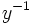# Right congruence

VIEW RELATED: Analogues of this | Variations of this | Opposites of this |
View a complete list of semi-basic definitions on this wiki

The notion of right congruence also makes sense in the more general context of a monoid. In fact, the same definition works.

## Definition

### Symbol-free definition

A right congruence on a group is an equivalence relation on the group with the property that the equivalence relation is preserved on right multiplication by any element of the group.

### Definition with symbols

A right congruence on a group$G$ is an equivalence relation$\equiv$ on$G$ such that:$a \equiv b \implies ac \equiv bc$

## Relation with other notions

The dual notion to right congruence is the notion of left congruence.

An equivalence relation is termed a congruence if it is both a left congruence and a right congruence.

## Correspondence between subgroups and right congruences

The following is true:

Right congruences are precisely the equivalence relations whose equivalence classes are the right cosets of a subgroup

### Proving that any right congruence gives right cosets

We first show that the equivalence class of the identity element is a subgroup. For this, we show the following three things:

• Identity elements:The identity element is equivalent to the identity element: This follows on account of the relation being reflexive
• Closure under multiplication: If$a,b \equiv e$, so is$ab$: The proof of this comes as follows. Suppose$a \equiv e$. Then$ab \equiv b$. We already know that$b \equiv e$. Hence, by the transitivity of$\equiv$, we have$ab \equiv e$.
• Closure under inverses: If$a \equiv e$, then we can right multiply both sides by$a^{-1}$ and obtain$e \equiv a^{-1}$

Let$H$ denote this subgroup. Then clearly, for any$x \in G$,$x \equiv hx$ (right multiplying$e \equiv h$ by$x$). Thus all the elements in the right coset of$H$ are in the same equivalence class as$x$.

Further, we can show that if$x \equiv y$, they must be in the same right coset. Suppose$x \equiv y$. Then, right multiply both sides by$y^{-1}$. This gives$xy^{-1} \equiv e$, hence$xy^{-1} \in H$ or$y \in Hx$.

### Proving that right cosets give a right congruence

This is more or less direct. PLACEHOLDER FOR INFORMATION TO BE FILLED IN: [SHOW MORE]